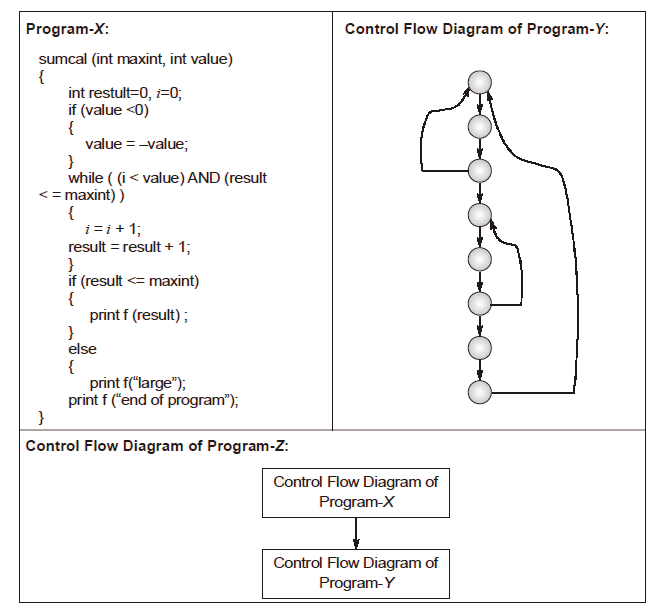Related Articles

# GATE | GATE-CS-2015 (Set 3) | Question 65

• Last Updated : 28 Jun, 2021

Consider three software items: Program-X, Control Flow Diagram of Program-Y and Control Flow Diagram of Program-Z as shown belowThe values of McCabe’s Cyclomatic complexity of Program-X, Program-Y and Program-Z respectively are
(A) 4, 4, 7
(B) 3, 4, 7
(C) 4, 4, 8
(D) 4, 3, 8

Explanation:

```The cyclomatic complexity of a structured program[a] is defined
with reference to the control flow graph of the program, a directed
graph containing the basic blocks of the program, with an edge
between two basic blocks if control may pass from the first to the
second. The complexity M is then defined as.

M = E − N + 2P,

where

E = the number of edges of the graph.
N = the number of nodes of the graph.
P = the number of connected components.

Source: http://en.wikipedia.org/wiki/Cyclomatic_complexity

For first program X, E = 11, N = 9, P = 1,  So M = 11-9+2*1 = 4
For second program Y, E = 10, N = 8, p = 1, So M = 10-8+2*1 = 4
For Third program X, E = 22, N = 17, p = 1, So M = 22-17+2*1 = 7```

Attention reader! Don’t stop learning now.  Practice GATE exam well before the actual exam with the subject-wise and overall quizzes available in GATE Test Series Course.

Learn all GATE CS concepts with Free Live Classes on our youtube channel.

My Personal Notes arrow_drop_up